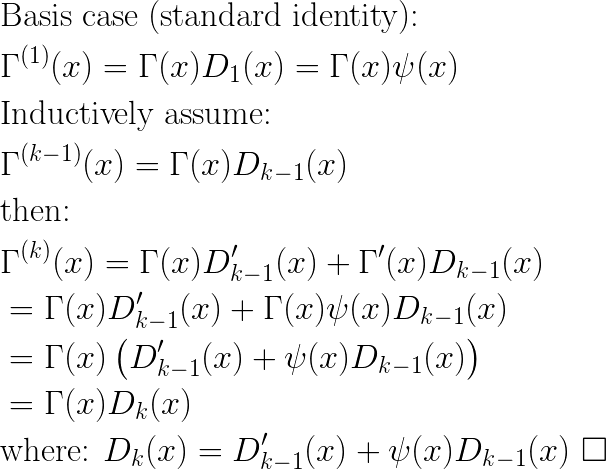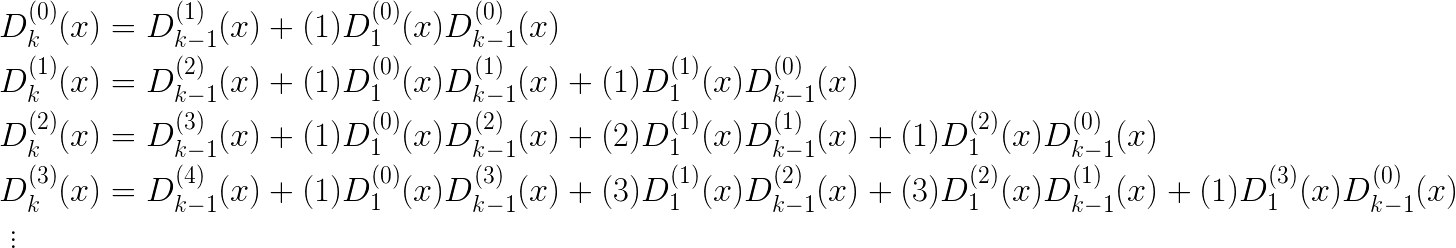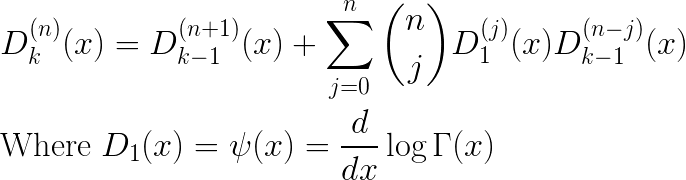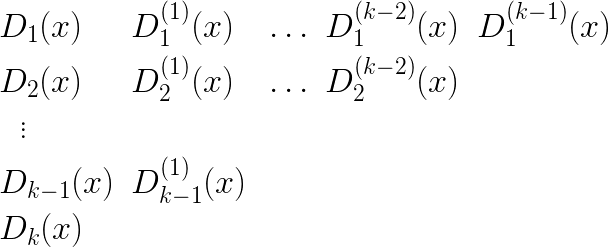In this post I’ll describe a simple algorithm to compute the kth derivatives of the Gamma function.

I’ll start by showing a simple recursion relation for these derivatives, and then gives its derivation. The kth derivative of Gamma(x) can be computed as follows:The recursive formula for the Dk functions has an easy inductive proof:Computing the next value Dk requires knowledge of Dk-1 but also derivative D’k-1. If we start expanding terms, we see the following:Continuing the process above it is not hard to see that we can continue expanding until we are left only with terms of D1(*)(x); that is, various derivatives of D1(x). Furthermore, each layer of substitutions adds an order to the derivatives, so that we will eventually be left with terms involving the derivatives of D1(x) up to the (k-1)th derivative. Note that these will all be successive orders of the polygamma function.

What we want, to do these computations systematically, is a formula for computing the nth derivative of a term Dk(x). Examining the first few such derivatives suggests a pattern:Generalizing from the above, we see that the formula for the nth derivative is:We are now in a position to fill in the triangular table of values, culminating in the value of Dk(x):As previously mentioned, the basis row of values D1(*)(x) are the polygamma functions where D1(n)(x) = polygamma(n)(x). The first two polygammas, order 0 and 1, are simply the digamma and trigamma functions, respectively, and are available with most numeric libraries. Computing the general polygamma is a project, and blog post, for another time, but the standard polynomial approximation for the digamma function can of course be differentiated… Happy Computing!